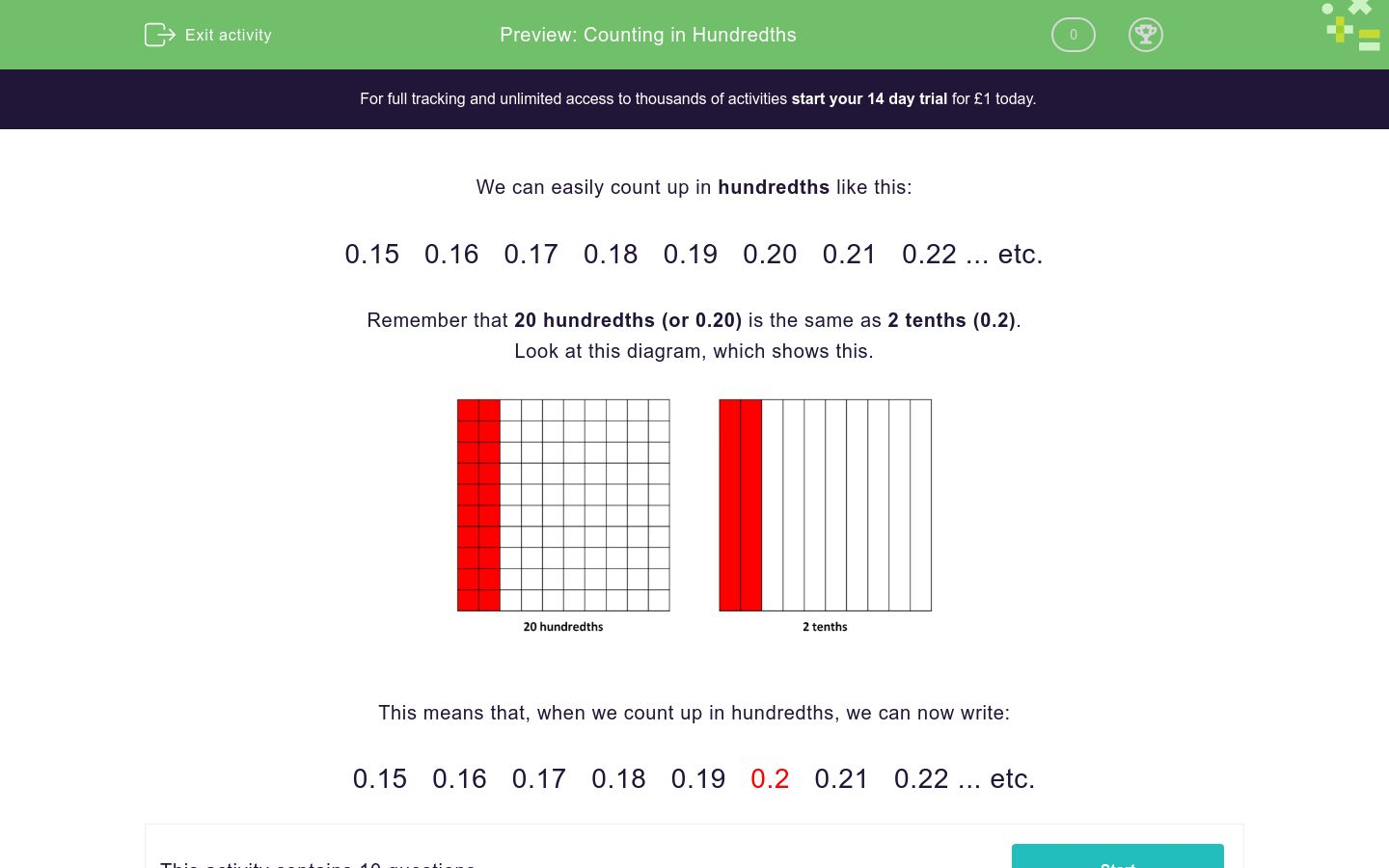# Counting in Hundredths

In this worksheet, students must count up and down in hundredths realising the connection between hundredths and tenths.Key stage:  KS 2

Curriculum topic:   Number: Fractions and Decimals

Curriculum subtopic:   Count in Hundredths

Difficulty level:### QUESTION 1 of 10

We can easily count up in hundredths like this:

0.15   0.16   0.17   0.18   0.19   0.20   0.21   0.22 ... etc.

Remember that 20 hundredths (or 0.20) is the same as 2 tenths (0.2).

Look at this diagram, which shows this.This means that, when we count up in hundredths, we can now write:

0.15   0.16   0.17   0.18   0.19   0.2   0.21   0.22 ... etc.

Count up in hundredths to work out the missing number.

2.45    2.46    2.472.49

What is the missing number?

Count up in hundredths to work out the missing number.

2.47    2.482.5    2.51

What is the missing number?

Count up in hundredths to work out the missing number.

4.48    4.494.51    4.52

What is the missing number?

(Write the number using tenths if you can.)

Count up in hundredths to work out the missing number.

13.713.72    13.73

What is the missing number?

Count up in hundredths to work out the missing number.7.9    7.91    7.92

What is the missing number?

Count up in hundredths to work out the missing number.

12.86    12.87    12.8812.9

What is the missing number?

Count up in hundredths to work out the missing number.

3.19    3.23.22

What is the missing number?

Count up in hundredths to work out the missing number.

16.78    16.7916.81    16.82

What is the missing number?

(Write the number using tenths if you can.)

Count up in hundredths to work out the missing number.

20.120.12

What is the missing number?

Count up in hundredths to work out the missing number.30.5    30.51    30.52

What is the missing number?

• Question 1

Count up in hundredths to work out the missing number.

2.45    2.46    2.472.49

What is the missing number?

2.48
EDDIE SAYS
2.45... 2.46... 2.47... 2.48... 2.49
• Question 2

Count up in hundredths to work out the missing number.

2.47    2.482.5    2.51

What is the missing number?

2.49
EDDIE SAYS
2.47... 2.48... 2.49... 2.5 or 2.50... 2.51
• Question 3

Count up in hundredths to work out the missing number.

4.48    4.494.51    4.52

What is the missing number?

(Write the number using tenths if you can.)

4.5
EDDIE SAYS
4.48... 4.49... 4.5 or 4.50... 4.51... 4.52
• Question 4

Count up in hundredths to work out the missing number.

13.713.72    13.73

What is the missing number?

13.71
EDDIE SAYS
13.7 or 13.70... 13.71... 13.72... 13.73
• Question 5

Count up in hundredths to work out the missing number.7.9    7.91    7.92

What is the missing number?

7.89
EDDIE SAYS
7.89... 7.9 or 7.90... 7.91... 7.92
• Question 6

Count up in hundredths to work out the missing number.

12.86    12.87    12.8812.9

What is the missing number?

12.89
EDDIE SAYS
12.86... 12.87... 12.88... 12.89... 12.9 or 12.90
• Question 7

Count up in hundredths to work out the missing number.

3.19    3.23.22

What is the missing number?

3.21
EDDIE SAYS
3.19... 3.2 or 3.20... 3.21... 3.22
• Question 8

Count up in hundredths to work out the missing number.

16.78    16.7916.81    16.82

What is the missing number?

(Write the number using tenths if you can.)

16.8
EDDIE SAYS
16.78... 16.79... 16.8 or 16.80... 16.81... 16.82
• Question 9

Count up in hundredths to work out the missing number.

20.120.12

What is the missing number?

20.11
EDDIE SAYS
20.1 or 20.10... 20.11... 20.12
• Question 10

Count up in hundredths to work out the missing number.30.5    30.51    30.52

What is the missing number?

30.49
EDDIE SAYS
30.49... 30.5 or 30.50... 30.51... 30.52
---- OR ----

Sign up for a £1 trial so you can track and measure your child's progress on this activity.

### What is EdPlace?

We're your National Curriculum aligned online education content provider helping each child succeed in English, maths and science from year 1 to GCSE. With an EdPlace account you’ll be able to track and measure progress, helping each child achieve their best. We build confidence and attainment by personalising each child’s learning at a level that suits them.

Get started